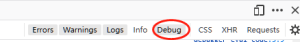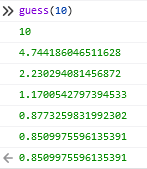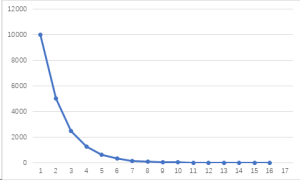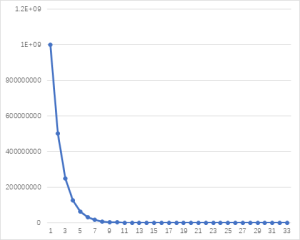# 牛顿法解方程-使用浏览器（Geogebra可选）做实验1．计算机问题求解的三类方法

。例如就解二元一次方程

a * x * x + b * x – c = 0

x1=（-b +（b^2-4*a*c)^1/2）/ (2*a)
x1=（-b -（b^2-4*a*c)^1/2）/ (2*a)

（在解存在的情况下，）我们把a,b,c代入公式就能求出方程的根。这种方法适用于 我们对于方程的规律全部都了解。对于计算机学生而言，这个公式相当于领域知识或者先验知识。

2． 牛顿法 算法步骤的几何解释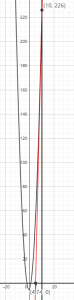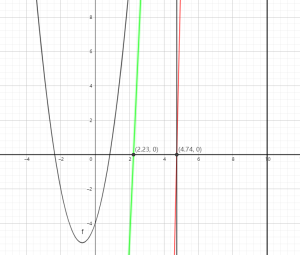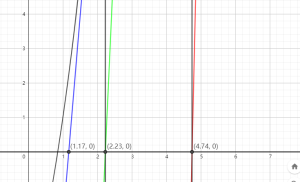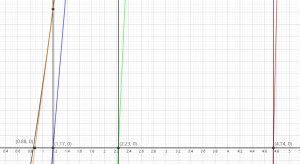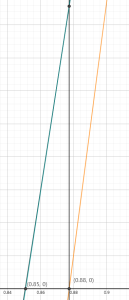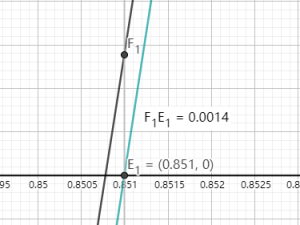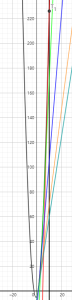3． 递归公式推导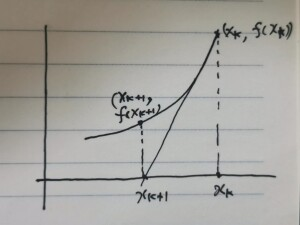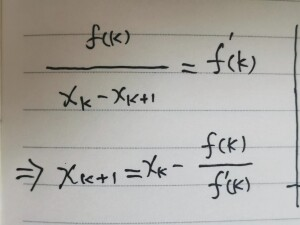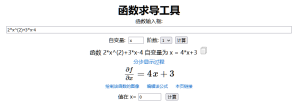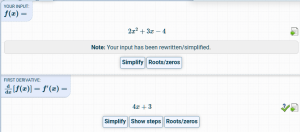3． JavaScript代码

```// In Geogebra
// f: y=2 x^(2)+3 x-4
// NSolve(0=2 x^(2)+3 x-4)
// = {x = -2.35, x = 0.85}
a = 2;
b = 3;
c = -4;
epsilon = 0.01;```

```function f(x)
{
return a*x*x+b*x+c;
}
function df(x) // derived function
{
return 2*a*x+b;
}```

```function guess(x)
{
return Math.abs(f(x)) <= epsilon ? x : guess( x - f(x)/df(x));
}```

`Math.abs(f(x)) <= epsilon ? x : guess( x - f(x)/df(x) )`

```guess(0); //0.8517236753856471
guess (-1); //-2.350915564067371```

4．验证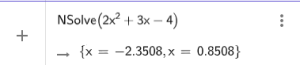4．递归有多快

```function guess(x)
{
console.debug(x);
return Math.abs(f(x)) <= epsilon ? x : guess( x - f(x)/df(x)
);
}```